# On orthogonal polynomials and quadrature rules related to the second kind of beta distribution

## Abstract

We consider a finite class of weighted quadratures with the weight function ${x}^{-2a}{\left(1+{x}^{2}\right)}^{-b}$ on $\left(-\mathrm{\infty },\mathrm{\infty }\right)$, which is valid only for finite values of n (the number of nodes). This means that classical Gauss-Jacobi quadrature rules cannot be considered for this class, because some restrictions such as $\left\{maxn\right\}\le a+b-1/2$, $a<1/2$, $b>0$ and ${\left(-1\right)}^{2a}=1$ must be satisfied for its orthogonality relation. Some analytic examples are given in this sense.

MSC:41A55, 65D30, 65D32.

## 1 Introduction

The differential equation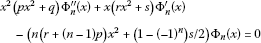(1)

was introduced in , and it was established that the symmetric polynomials

$\begin{array}{rcl}{\mathrm{\Phi }}_{n}\left(x\right)& =& {S}_{n}\left(\begin{array}{cc}r& s\\ p& q\end{array}|x\right)\\ =& \sum _{k=0}^{\left[n/2\right]}\left(\begin{array}{c}k\end{array}\right)\left(\prod _{i=0}^{\left[n/2\right]-\left(k+1\right)}\frac{\left(2i+{\left(-1\right)}^{n+1}+2\left[n/2\right]\right)p+r}{\left(2i+{\left(-1\right)}^{n+1}+2\right)q+s}\right){x}^{n-2k}\end{array}$
(2)

are a basis solution of it. If this equation is written in a self-adjoint form, then the first-order equation

$x\frac{d}{dx}\left(\left(p{x}^{2}+q\right)W\left(x\right)\right)=\left(r{x}^{2}+s\right)W\left(x\right)$
(3)

would appear. The solution of equation (3) is known as an analogue of Pearson distributions family and can be indicated as

$W\left(\begin{array}{cc}r& s\\ p& q\end{array}|x\right)=exp\left(\int \frac{\left(r-2p\right){x}^{2}+s}{x\left(p{x}^{2}+q\right)}\phantom{\rule{0.2em}{0ex}}dx\right).$
(4)

There are four main sub-classes of distributions family (4) (and consequently, sub-solutions of equation (3)) whose explicit probability density functions are, respectively, as follows: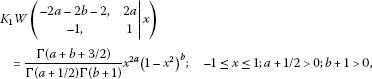(5)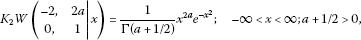(6)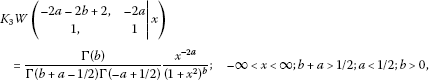(7)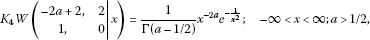(8)

where ${K}_{i}$; $i=1,2,3,4$ play the normalizing constant role.

Clearly, the value of distribution vanishes at $x=0$ in each of the above mentioned four cases, i.e., $W\left(p,q,r,s;0\right)=0$ for $s\ne 0$.

As a special case of (4), let us consider the values $p=1$, $q=1$, $r=-2a-2b+2$ and $s=-2a$ corresponding to distribution (7) and replace them in equation (1) to get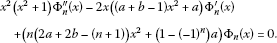(9)

By solving equation (9), the polynomial solution of monic type is derived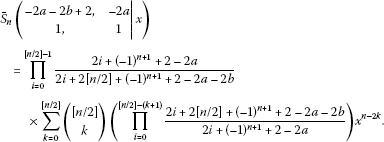(10)

According to , these polynomials are finitely orthogonal with respect to the second kind of beta weight function ${x}^{-2a}{\left(1+{x}^{2}\right)}^{-b}$ on $\left(-\mathrm{\infty },\mathrm{\infty }\right)$ if and only if $\left\{maxn\right\}\le a+b-1/2$, i.e., we have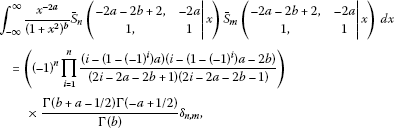(11)

if $m,n=0,1,\dots$ , $N\le a+b-1/2$, where $N=max\left\{m,n\right\}$, ${\delta }_{n,m}=\left\{\begin{array}{cc}0\hfill & \left(n\ne m\right),\hfill \\ 1\hfill & \left(n=m\right),\hfill \end{array}$ $a<1/2$, $b>0$ and ${\left(-1\right)}^{2a}=1$. Moreover, they satisfy a three-term recurrence relation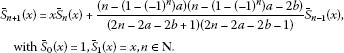(12)

The orthogonality property (11) shows that the polynomials ${\overline{S}}_{n}\left(1,1,-2a-2b+2,-2a;x\right)$ are a suitable tool to finitely approximate the functions that satisfy the Dirichlet conditions .

For example, if $N=\left\{maxn\right\}=3$, $a+b\ge 7/2$, $a<1/2$, $b>0$ and ${\left(-1\right)}^{2a}=1$ in (10), then the arbitrary function $f\left(x\right)$ can be approximated as

$f\left(x\right)\cong \sum _{m=0}^{3}{B}_{m}{\overline{S}}_{m}\left(1,1,-2a-2b+2,-2a;x\right),$
(13)

where

$\begin{array}{rcl}{B}_{m}& =& \left({\left(-1\right)}^{m}\prod _{i=1}^{m}\frac{\left(i-\left(1-{\left(-1\right)}^{i}\right)a\right)\left(i-\left(1-{\left(-1\right)}^{i}\right)a-2b\right)}{\left(2i-2a-2b+1\right)\left(2i-2a-2b-1\right)}\right)\frac{\mathrm{\Gamma }\left(b+a-1/2\right)\mathrm{\Gamma }\left(-a+1/2\right)}{\mathrm{\Gamma }\left(b\right)}\\ ×{\int }_{-\mathrm{\infty }}^{\mathrm{\infty }}\frac{{x}^{-2a}}{{\left(1+{x}^{2}\right)}^{b}}{\overline{S}}_{m}\left(\begin{array}{cc}-2a-2b+2& -2a\\ 1& 1\end{array}|x\right)f\left(x\right)\phantom{\rule{0.2em}{0ex}}dx.\end{array}$
(14)

This means that the finite set ${\left\{{\overline{S}}_{i}\left(1,1,-2a-2b+2,-2a;x\right)\right\}}_{i=0}^{3}$ is a basis space for all polynomials of degree at most three, i.e., for $f\left(x\right)={a}_{3}{x}^{3}+{a}_{2}{x}^{2}+{a}_{1}x+{a}_{0}$, the approximation (13) is exact. This matter helps us to state the application of symmetric orthogonal polynomials (10) in weighted quadrature rules .

## 2 Application of ${\overline{S}}_{n}\left(1,1,-2a-2b+2,-2a;x\right)$ in quadrature rules

Consider the general form of a weighted quadrature

${\int }_{\alpha }^{\beta }w\left(x\right)f\left(x\right)\phantom{\rule{0.2em}{0ex}}dx=\sum _{i=1}^{n}{w}_{i}f\left({x}_{i}\right)+\sum _{k=1}^{m}{v}_{k}f\left({z}_{k}\right)+{R}_{n,m}\left[f\right],$
(15)

where $w\left(x\right)$ is a positive function on $\left[\alpha ,\beta \right]$; ${\left\{{w}_{i}\right\}}_{i=1}^{n}$, ${\left\{{v}_{k}\right\}}_{k=1}^{m}$ are unknown coefficients; ${\left\{{x}_{i}\right\}}_{i=1}^{n}$ are unknown nodes; ${\left\{{z}_{k}\right\}}_{k=1}^{m}$ are pre-determined nodes [7, 8]; and finally, the residue ${R}_{n,m}\left[f\right]$ is determined (see, e.g., ) by

${R}_{n,m}\left[f\right]=\frac{{f}^{\left(2n+m\right)}\left(\xi \right)}{\left(2n+m\right)!}{\int }_{\alpha }^{\beta }w\left(x\right)\prod _{k=1}^{m}\left(x-{z}_{k}\right)\prod _{i=1}^{n}{\left(x-{x}_{i}\right)}^{2}\phantom{\rule{0.2em}{0ex}}dx;\phantom{\rule{1em}{0ex}}\alpha <\xi <\beta .$
(16)

It can be shown in (15) that ${R}_{n,m}\left[f\right]=0$ for any linear combination of the sequence $\left\{1,x,\dots ,{x}^{2n+m+1}\right\}$ if and only if ${\left\{{x}_{i}\right\}}_{i=1}^{n}$ are the roots of orthogonal polynomials of degree n with respect to the weight function $w\left(x\right)$, and ${\left\{{z}_{k}\right\}}_{k=1}^{m}$ belong to $\left[\alpha ,\beta \right]$; see  for more details. Also, it is proved that to derive ${\left\{{w}_{i}\right\}}_{i=1}^{n}$ in (15), when $m=0$, it is not required to solve the following linear system of order $n×n$:

(17)

Rather, one can directly use the relation

(18)

in which ${\stackrel{ˆ}{P}}_{i}\left(x\right)$ is the orthonormal polynomial of ${P}_{i}\left(x\right)$, i.e.,

${\stackrel{ˆ}{P}}_{i}\left(x\right)={\left({\int }_{\alpha }^{\beta }w\left(x\right){P}_{i}^{2}\left(x\right)\phantom{\rule{0.2em}{0ex}}dx\right)}^{-1/2}{P}_{i}\left(x\right).$
(19)

Now, by noting that the symmetric polynomials (10) are finitely orthogonal with respect to the weight function $W\left(x,a,b\right)={x}^{-2a}{\left(1+{x}^{2}\right)}^{-b}$ on the real line, we consider the following finite class of quadrature rules: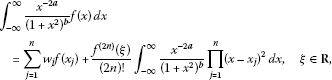(20)

where ${x}_{j}$ are the roots of polynomials ${\overline{S}}_{n}\left(1,1,-2a-2b+2,-2a;x\right)$ and ${w}_{j}$ are calculated by

(21)

### 2.1 An important remark

The change of variable $x={t}^{-1/2}{\left(1-t\right)}^{1/2}$ in the left-hand side of (20) first changes the interval $\left(-\mathrm{\infty },\mathrm{\infty }\right)$ to $\left[0,1\right]$ such that we have

${\int }_{-\mathrm{\infty }}^{\mathrm{\infty }}\frac{{x}^{-2a}}{{\left(1+{x}^{2}\right)}^{b}}f\left(x\right)\phantom{\rule{0.2em}{0ex}}dx={\int }_{0}^{1}{t}^{a+b-\frac{3}{2}}{\left(1-t\right)}^{-a-\frac{1}{2}}f\left(\sqrt{\frac{1}{t}-1}\right)\phantom{\rule{0.2em}{0ex}}dt.$
(22)

As the right-hand integral of (22) shows, the shifted Jacobi weight function ${\left(1-x\right)}^{u}{x}^{v}$ has appeared for $u=-a-1/2$ and $v=a+b-3/2$. Hence, the shifted Gauss-Jacobi quadrature rule [6, 9] with the special parameters $u=-a-1/2$ and $v=a+b-3/2$ can also be applied for estimating (22). This procedure eventually changes (20) into the form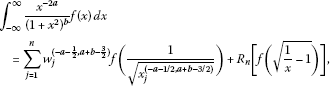(23)

where ${x}_{j}^{\left(-a-1/2,a+b-3/2\right)}$ are the zeros of shifted Jacobi polynomials ${P}_{n,+}^{\left(-a-1/2,a+b-3/2\right)}\left(x\right)$ on $\left[0,1\right]$. But, there is the main problem for the formula (23). From (16), it is generally known that the residue of quadrature rules depends on ${f}^{\left(2n\right)}\left(\xi \right)$; $\alpha <\xi <\beta$. Therefore, by noting (23), we should have

$\frac{{d}^{2n}f\left(\sqrt{{x}^{-1}-1}\right)}{d{x}^{2n}}=\sum _{i=0}^{2n}{\phi }_{i}\left(x\right){f}^{\left(i\right)}\left(\sqrt{{x}^{-1}-1}\right),$
(24)

where ${\phi }_{i}$ are real functions to be computed and ${f}^{\left(i\right)}$, $i=0,1,2,\dots ,2n$ are the successive derivatives of the function f. On the other hand, the function f cannot be in the form of an arbitrary polynomial in order that the right-hand side of (24) becomes zero. In other words, the formula (23) cannot be exact for all elements of the basis $f\left(x\right)={x}^{j}$; $j=0,1,2,\dots ,2n-1$. This is the main disadvantage of using (23), which shows the importance of the polynomials (10) in estimating a class of weighted quadrature rules . The following examples clarify this remark.

Example 1

${\int }_{-\mathrm{\infty }}^{\mathrm{\infty }}{x}^{-2a}{\left(1+{x}^{2}\right)}^{-b}f\left(x\right)\phantom{\rule{0.2em}{0ex}}dx\cong {w}_{1}f\left({x}_{1}\right)+{w}_{2}f\left({x}_{2}\right),$
(25)

in which $a+b\ge 5/2$, $a<1/2$, $b>0$ and ${\left(-1\right)}^{2a}=1$. According to the explained comments, (25) must be exact for all elements of the basis $f\left(x\right)=\left\{1,x,{x}^{2},{x}^{3}\right\}$ if and only if ${x}_{1}$, ${x}_{2}$ are two roots of ${\overline{S}}_{2}\left(1,1,-2a-2b+2,-2a;x\right)$. As a particular sample, let us take $a=0$ and $b=3$. Then (25) is reduced to

${\int }_{-\mathrm{\infty }}^{\mathrm{\infty }}\frac{1}{{\left(1+{x}^{2}\right)}^{3}}f\left(x\right)\phantom{\rule{0.2em}{0ex}}dx\cong {w}_{1}f\left(\frac{\sqrt{3}}{3}\right)+{w}_{2}f\left(-\frac{\sqrt{3}}{3}\right),$
(26)

in which $\sqrt{3}/3$ and $-\sqrt{3}/3$ are zeros of ${\overline{S}}_{2}\left(1,1,-4,0;x\right)$ and ${w}_{1}$, ${w}_{2}$ are computed by solving the linear system

${w}_{1}+{w}_{2}={\int }_{-\mathrm{\infty }}^{\mathrm{\infty }}{\left(1+{x}^{2}\right)}^{-3}\phantom{\rule{0.2em}{0ex}}dx=\frac{3}{8}\pi ,\phantom{\rule{2em}{0ex}}\frac{\sqrt{3}}{3}\left({w}_{1}-{w}_{2}\right)={\int }_{-\mathrm{\infty }}^{\mathrm{\infty }}x{\left(1+{x}^{2}\right)}^{-3}\phantom{\rule{0.2em}{0ex}}dx=0.$
(27)

After deriving ${w}_{1}$, ${w}_{2}$ in (27), the complete form of (26) would be

${\int }_{-\mathrm{\infty }}^{\mathrm{\infty }}\frac{1}{{\left(1+{x}^{2}\right)}^{3}}f\left(x\right)\phantom{\rule{0.2em}{0ex}}dx=\frac{3\pi }{16}\left(f\left(\frac{\sqrt{3}}{3}\right)+f\left(-\frac{\sqrt{3}}{3}\right)\right)+{R}_{2}\left[f\right],$
(28)

where

${R}_{2}\left[f\right]=\frac{{f}^{\left(4\right)}\left(\xi \right)}{4!}{\int }_{-\mathrm{\infty }}^{\mathrm{\infty }}\frac{1}{{\left(1+{x}^{2}\right)}^{3}}{\left({\overline{S}}_{2}\left(\begin{array}{cc}-4& 0\\ 1& 1\end{array}|x\right)\right)}^{2}\phantom{\rule{0.2em}{0ex}}dx=\frac{\pi }{72}{f}^{\left(4\right)}\left(\xi \right),\phantom{\rule{1em}{0ex}}\xi \in \mathbf{R}.$
(29)

Relation (28) shows that it is exact for any arbitrary polynomial of degree at most three.

Example 2 To have a three-point formula of type (20), first we should note that the conditions $a+b\ge 7/2$, $a<1/2$, $b>0$ and ${\left(-1\right)}^{2a}=1$ must be satisfied. For instance, if $a=-1$ and $b=5$, then after some computations, the related formula takes the form

${\int }_{-\mathrm{\infty }}^{\mathrm{\infty }}\frac{{x}^{2}}{{\left(1+{x}^{2}\right)}^{5}}f\left(x\right)\phantom{\rule{0.2em}{0ex}}dx=\frac{\pi }{1,280}\left(9f\left(\sqrt{\frac{5}{3}}\right)+32f\left(0\right)+9f\left(-\sqrt{\frac{5}{3}}\right)\right)+{R}_{3}\left[f\right],$
(30)

where

${R}_{3}\left[f\right]=\frac{{f}^{\left(6\right)}\left(\xi \right)}{6!}{\int }_{-\mathrm{\infty }}^{\mathrm{\infty }}\frac{{x}^{2}}{{\left(1+{x}^{2}\right)}^{5}}{\left({\overline{S}}_{3}\left(\begin{array}{cc}-6& 2\\ 1& 1\end{array}|x\right)\right)}^{2}\phantom{\rule{0.2em}{0ex}}dx=\frac{5\pi }{3,456}{f}^{\left(6\right)}\left(\xi \right),\phantom{\rule{1em}{0ex}}\xi \in \mathbf{R},$
(31)

and ${x}_{1}=\sqrt{5/3}$, ${x}_{2}=0$, ${x}_{3}=-\sqrt{5/3}$ are the roots of ${\overline{S}}_{3}\left(1,1,-6,2;x\right)={x}^{3}-\left(5/3\right)x$.

Example 3 To derive a four-point formula of type (20), first the conditions $a+b\ge 9/2$, $a<1/2$, $b>0$ and ${\left(-1\right)}^{2a}=1$ must be satisfied. For example, if $a=0$ and $b=6$, then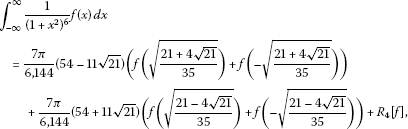(32)

where

$\begin{array}{rcl}{R}_{4}\left[f\right]& =& \frac{{f}^{\left(8\right)}\left(\xi \right)}{8!}{\int }_{-\mathrm{\infty }}^{\mathrm{\infty }}\frac{1}{{\left(1+{x}^{2}\right)}^{6}}{\left({\overline{S}}_{4}\left(\begin{array}{cc}-10& 0\\ 1& 1\end{array}|x\right)\right)}^{2}\phantom{\rule{0.2em}{0ex}}dx\\ =& \frac{\pi }{2,822,400}{f}^{\left(8\right)}\left(\xi \right),\phantom{\rule{1em}{0ex}}\xi \in \mathbf{R}.\end{array}$
(33)

This formula is exact for all elements of the basis $f\left(x\right)={x}^{j}$; $j=0,1,2,\dots ,7$ and its nodes are the roots of ${\overline{S}}_{4}\left(1,0,-8,2;x\right)={x}^{4}-\left(6/5\right){x}^{2}+3/35$.

Tables 1-3 show some numerical examples related to three given examples.

## References

1. Masjed-Jamei M: A basic class of symmetric orthogonal polynomials using the extended Sturm-Liouville theorem for symmetric functions. J. Math. Anal. Appl. 2007, 325: 753–775. 10.1016/j.jmaa.2006.02.007

2. Masjed-Jamei M: Three finite classes of hypergeometric orthogonal polynomials and their application in functions approximation. Integral Transforms Spec. Funct. 2002, 13: 169–190. 10.1080/10652460212898

3. Masjed-Jamei M, Srivastava HM: An integral expansion for analytic functions based upon the remainder values of the Taylor series expansions. Appl. Math. Lett. 2009, 22: 406–411. 10.1016/j.aml.2008.03.030

4. Masjed-Jamei M, Srivastava HM: Application of a new integral expansion for solving a class of functional equations. Appl. Math. Lett. 2010, 23: 421–425. 10.1016/j.aml.2009.11.010

5. Masjed-Jamei M, Hussain N: More results on a functional generalization of the Cauchy-Schwarz inequality. J. Inequal. Appl. 2012., 2012: Article ID 239

6. Davis R, Rabinowitz P: Methods of Numerical Integration. 2nd edition. Academic Press, New York; 1984.

7. Gautschi W: Construction of Gauss-Christoffel quadrature formulas. Math. Comput. 1968, 22: 251–270. 10.1090/S0025-5718-1968-0228171-0

8. Krylov VI: Approximate Calculation of Integrals. Macmillan Co., New York; 1962.

9. Masjed-Jamei M, Kutbi MA, Hussain N: Some new estimates for the error of Simpson integration rule. Abstr. Appl. Anal. 2012., 2012: Article ID 239695

10. Stoer J, Bulirsch R: Introduction to Numerical Analysis. 2nd edition. Springer, New York; 1993.

## Acknowledgements

Dedicated to Professor Hari M Srivastava.

The work of the first author is supported by a grant from Iran National Science Foundation and the work of the second author has been supported by the Deanship of Scientific Research (DSR) at King Abdulaziz University (KAU) of Jeddah.

## Author information

Authors

### Corresponding author

Correspondence to Nawab Hussain.

### Competing interests

The authors declare that they have no competing interests.

### Authors’ contributions

Both authors contributed equally and significantly in writing this article. Both authors read and approved the final manuscript.

## Rights and permissions

Reprints and Permissions

Masjed-Jamei, M., Hussain, N. On orthogonal polynomials and quadrature rules related to the second kind of beta distribution. J Inequal Appl 2013, 157 (2013). https://doi.org/10.1186/1029-242X-2013-157